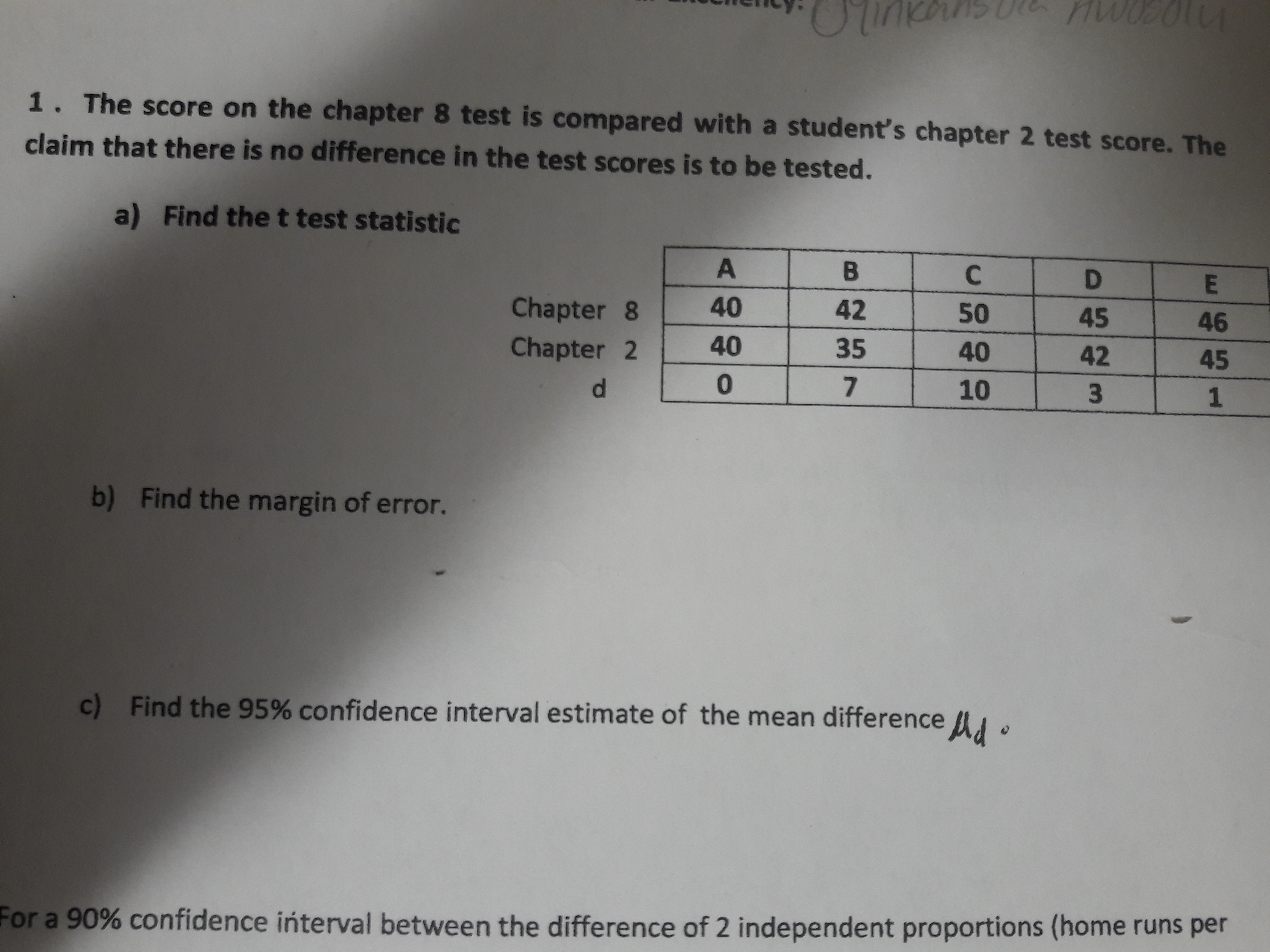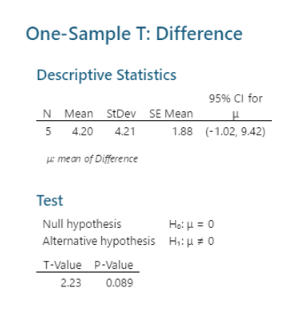# Ciency:1. The score on the chapter 8 test is compared with a student's chapter 2 test score. Theclaim that there is no difference in the test scores is to be tested.a)Find thet test statisticChapter 84042504546Chapter 235404245d.010b) Find the margin of error.c) Find the 95% confidence interval estimate of the mean difference .For a 90% confidence interval between the difference of 2 independent proportions (home runs per

Question
1 viewshelp_outlineImage TranscriptioncloseCiency: 1. The score on the chapter 8 test is compared with a student's chapter 2 test score. The claim that there is no difference in the test scores is to be tested. a) Find thet test statistic Chapter 8 40 42 50 45 46 Chapter 2 35 40 42 45 d. 0 10 b) Find the margin of error. c) Find the 95% confidence interval estimate of the mean difference . For a 90% confidence interval between the difference of 2 independent proportions (home runs per fullscreen
check_circle

Step 1

(a)

Step by step procedure to obtain the test statistic using MINITAB software is given below:

• Choose Stat > Basic Statistics > 1-Sample t.
• In One or more samples, each in a column, select Difference.
• Check Options, enter Confidence level as 95.
• In Perform Hypothesis test, enter 0 Under Hypothesized mean.
• Choose Mean ≠ Hypothesized mean in alternative.
• Click OK in all dialogue boxes.

Output using the MINITAB software is given below:help_outlineImage TranscriptioncloseOne-Sample T: Difference Descriptive Statistics 95% CI for N Mean StDev SE Mean 5 4.20 4.21 1.88 (-1.02, 9.42) e mean of Difference Test Null hypothesis Alternative hypothesis Heμ- 0 Hi: u = 0 T-Value P-Value 2.23 0.089 fullscreen
Step 2

From the MINITAB output, the value of test statistic is 2.33.

(b)

The degrees of freedom is obtained as follows:

Step 3

The critical value is obtai...

### Want to see the full answer?

See Solution

#### Want to see this answer and more?

Solutions are written by subject experts who are available 24/7. Questions are typically answered within 1 hour.*

See Solution
*Response times may vary by subject and question.
Tagged in

### Statistics